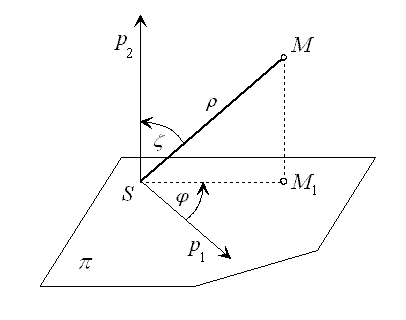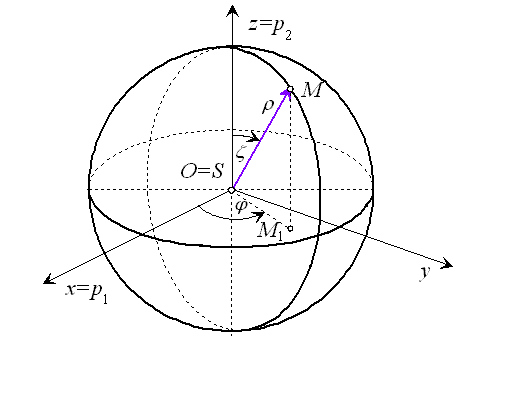# Spherical coordinates

Spherical coordinate system in the space is determined by the plane $\pi$, in which the oriented half-line ${\stackrel{\to }{p}}_{1}$ with the start point S and the anti-clockwise revolution are chosed, and by the oriented half-line ${\stackrel{\to }{p}}_{2}$ with the start point S and perpendicular to $\pi$.

In the spherical coordinate system $\left(S,\phantom{\rule{2mm}{2mm}}\rho ,\phantom{\rule{2mm}{2mm}}\varphi ,\phantom{\rule{2mm}{2mm}}\zeta \right)$ any point M in the space, which is not on line p coinciding with the half-line ${\stackrel{\to }{p}}_{2}$, can be attached a unique triple of real numbers $\rho ,\phantom{\rule{2mm}{2mm}}\varphi$ and $\zeta$, with a clear geometric interpretationFigure 1: Spherical coordinate system

1. $\rho =|SM|$, so $\rho$ is the distance of points M and S
2. $\varphi =|\sphericalangle \left({\stackrel{\to }{p}}_{1},\phantom{\rule{mediummathspace}{0.2em}}\stackrel{\to }{S{M}_{1}}\right)|$, thus $\varphi$ is size of the oriented angle with the vertex in point S, the first arm is formed by half-line $\stackrel{\to }{{p}_{1}}$, the second arm by half-line ${\stackrel{\to }{SM}}_{1}$, while ${M}_{1}$ is the orthographic view of M in the plane $\pi$
3. $\zeta =|\sphericalangle \left({\stackrel{\to }{p}}_{2},\phantom{\rule{2mm}{2mm}}\stackrel{\to }{SM}\right)|$, thus $\zeta \phantom{\rule{2mm}{2mm}}$is the size of angle formed by $\stackrel{\to }{{p}_{2}}$ and $\stackrel{\to }{SM}$.

An ordered triple of real numbers $\left(\rho ,\varphi ,\zeta \right)$ form spherical coordinates of the point M, for which holds $\rho \in \left(0,\infty \right)$, $\varphi \in \left[0,\phantom{\rule{2mm}{2mm}}2\pi \right)$, or $\varphi \in \left(-\pi ,\pi \right],\phantom{\rule{2mm}{2mm}}\zeta \in \left[0,\pi \right)$.

If point M is located on line p, its spherical coordinates are in the form of a triple

 $\left(\rho ,\varphi ,\zeta \right)$

where $\varphi$ is and arbitrary number, and $\zeta =0$ for points M located on half-line $\stackrel{\to }{{p}_{2}}$ , while $\zeta =\pi$ in the opposite case.Figure 2: Relation between spherical and Cartesian coordinates

Spherical coordinate system $\left(S,\phantom{\rule{2mm}{2mm}}\rho ,\phantom{\rule{2mm}{2mm}}\varphi ,\phantom{\rule{2mm}{2mm}}\zeta \right)$ is related (conjugate) to the Cartesian orthogonal coordinate system $\left(O,\phantom{\rule{2mm}{2mm}}x,\phantom{\rule{2mm}{2mm}}y,\phantom{\rule{2mm}{2mm}}z\right)$ in the space, iff

1. plane $\pi$ determining the spherical coordinate system $\left(S,\phantom{\rule{2mm}{2mm}}\rho ,\phantom{\rule{2mm}{2mm}}\varphi ,\phantom{\rule{2mm}{2mm}}\zeta \right)$ coincides with the plane $xy\phantom{\rule{2mm}{2mm}}$of the Cartesian coordinate system $\left(O,\phantom{\rule{2mm}{2mm}}x,\phantom{\rule{2mm}{2mm}}y,\phantom{\rule{2mm}{2mm}}z\right)$
2. point S coincides with the origin O and oriented half-line $\stackrel{\to }{{p}_{1}}$ coincides with the positive part of the axis x
3. oriented half-line $\stackrel{\to }{{p}_{2}}$ coincides with the positive part of the axis z.

If $\left(x,\phantom{\rule{2mm}{2mm}}y,\phantom{\rule{2mm}{2mm}}z\right)$ are Cartesian coordinates and $\left(\rho ,\phantom{\rule{2mm}{2mm}}\varphi ,\phantom{\rule{2mm}{2mm}}\zeta \right)$ are spherical coordinates of point M not located on the coordinate axis z, their relation can be represented by the following equations

 $x=|S{M}_{1}|\mathrm{cos}\phantom{\rule{mediummathspace}{0.2em}}\varphi$
 $y=|S{M}_{1}|\mathrm{sin}\phantom{\rule{mediummathspace}{0.2em}}\varphi$
 $z=\rho \mathrm{cos}\phantom{\rule{mediummathspace}{0.2em}}\zeta$

and since $|S{M}_{1}|=\rho \mathrm{sin}\phantom{\rule{2mm}{2mm}}\zeta$ , it is valid

 $x=\rho \mathrm{cos}\phantom{\rule{mediummathspace}{0.2em}}\varphi \phantom{\rule{mediummathspace}{0.2em}}\mathrm{sin}\phantom{\rule{mediummathspace}{0.2em}}\zeta$
 $y=\rho \mathrm{sin}\phantom{\rule{mediummathspace}{0.2em}}\varphi \phantom{\rule{mediummathspace}{0.2em}}\mathrm{sin}\phantom{\rule{mediummathspace}{0.2em}}\zeta$
 $z=\rho \mathrm{cos}\phantom{\rule{mediummathspace}{0.2em}}\zeta$

from which the spherical coordinates can be expressed

 $\rho =\sqrt{{x}^{2}+{y}^{2}+{z}^{2}}$
 $\varphi =\mathrm{arccos}\phantom{\rule{mediummathspace}{0.2em}}\frac{x}{\sqrt{{x}^{2}+{y}^{2}}},\phantom{\rule{2mm}{2mm}}y\geqq 0$
 $\varphi =2\pi -\mathrm{arccos}\phantom{\rule{mediummathspace}{0.2em}}\frac{x}{\sqrt{{x}^{2}+{y}^{2}}},\phantom{\rule{2mm}{2mm}}y<0$
 $\zeta =\mathrm{arccos}\phantom{\rule{mediummathspace}{0.2em}}\frac{x}{\sqrt{{x}^{2}+{y}^{2}+{z}^{2}}}$

Set of all points in the space with the constant first spherical coordinate $\rho =a>0$ is sphere with centre in the origin of the coordinate system S and radius a.